### Confidence Intervals for Parameters

#### Likelihood Ratio-Based Confidence Intervals

PROC GENMOD produces likelihood ratio-based confidence intervals, also known as profile likelihood confidence intervals, for parameter estimates for generalized linear models. These are not computed for GEE models, since there is no likelihood for this type of model. Suppose that the parameter vector isand that you want a confidence interval for. The profile likelihood function foris defined aswhereis the vectorwith the jth element fixed atand l is the log-likelihood function. Ifis the log likelihood evaluated at the maximum likelihood estimate, then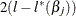has a limiting chi-square distribution with one degree of freedom ifis the true parameter value. A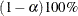confidence interval for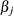iswhereis the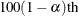percentile of the chi-square distribution with one degree of freedom. The endpoints of the confidence interval can be found by solving numerically for values ofthat satisfy equality in the preceding relation. PROC GENMOD solves this by starting at the maximum likelihood estimate of. The log-likelihood function is approximated with a quadratic surface, for which an exact solution is possible. The process is iterated until convergence to an endpoint is attained. The process is repeated for the other endpoint.

Convergence is controlled by the CICONV= option in the MODEL statement. Supposeis the number specified in the CICONV= option. The default value ofis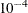. Let the parameter of interest be, and define, the unit vector with a 1 in position j and 0s elsewhere. Convergence is declared on the current iteration if the following two conditions are satisfied: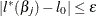where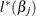,, andare the log likelihood, the gradient, and the Hessian evaluated at the current parameter vector andis a constant computed by the procedure. The first condition for convergence means that the log-likelihood function must be withinof the correct value, and the second condition means that the gradient vector must be proportional to the restriction vector.

When you specify the LRCI option in the MODEL statement, PROC GENMOD computes profile likelihood confidence intervals for all parameters in the model, including the scale parameter, if there is one. The interval endpoints are displayed in a table as well as the values of the remaining parameters at the solution.

#### Wald Confidence Intervals

You can request that PROC GENMOD produce Wald confidence intervals for the parameters. The ()100% Wald confidence interval for a parameteris defined as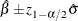whereis thepercentile of the standard normal distribution,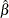is the parameter estimate, andis the estimate of its standard error.